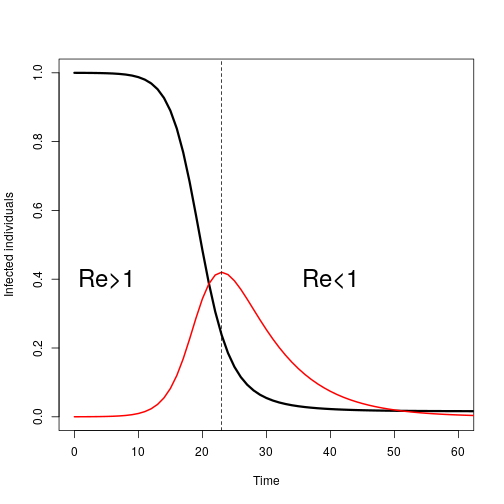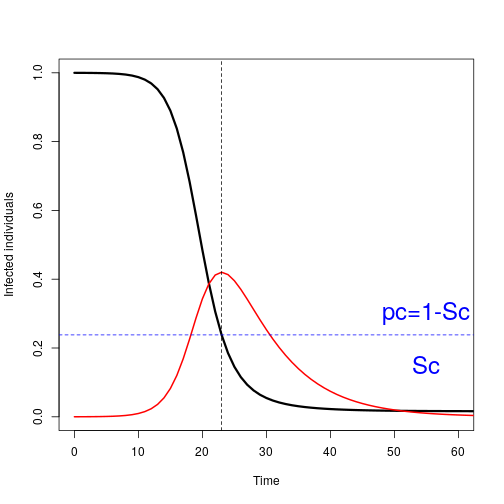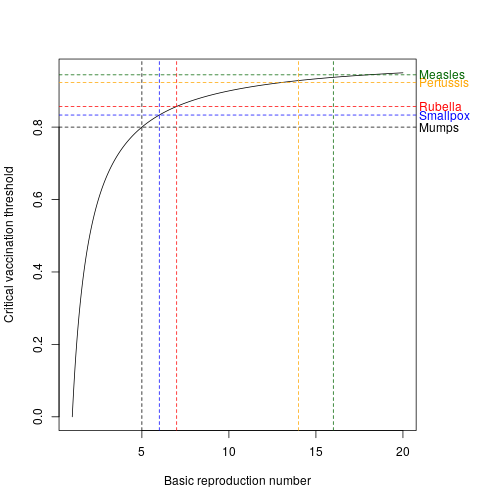# Epidemic interventions

John M. Drake

### Anatomy of an epidemic• Initial growth of the epidemic is exponential
• Susceptible depletion results in departure from the basic reproduction number
• Define effective reproduction number and denote $$R_e$$
• $$R_e$$ scales with the proportion of susceptibles in the population ($$S=X/N \implies R_e = R_0 \times S$$)
• This implies that there is a critical susceptible population size, $$S_c$$ for the pathogen to invade

### Vaccination

Recall that $$R_e = R_0 X/N$$

This implies that $$S_c = 1/R_0$$

The complement of $$S_c$$ is the critical vaccination proportion: $$p_c = 1-S_c = 1-\frac{1}{R_0}$$

A correction is needed for imperfect vaccines (e.g. SARS-CoV-2): $$p_c = \frac{1}{\epsilon}\left({1-\frac{1}{R_0}}\right)$$ where $$\epsilon$$ is the vaccine effectiveness at preventing transmissible infection.

### Vaccination### Vaccination

We can obtain the same result by inspection of the equation for $$dY/dt$$

$\frac{dY}{dt} = \beta X \frac{Y}{N} - \gamma Y$

Set $$dY/dt < 0$$ and solve

\begin{align} \frac{dY}{dt} = \beta X \frac{Y}{N} - \gamma Y &< 0 \\ \beta \frac{X}{N} &< \gamma \\ \frac{X}{N} &< \frac{\gamma}{\beta} = \frac{1}{R_0} \end{align}

### Vaccination thresholds### Population ("Herd") Immunity

Due to the dependency upon social contact for transmission, an individual is afforded a degree of protection via the immunity of others in the population.

If contacts have been vaccinated, the probability of acquiring the disease is lower

Implication: Not everyone in a population needs to be immune to eradicate an infections disease

The extent of vaccination effort required is determined by $$R_0$$.

“Cocooning” strategies

### A model for pediatric immunization

Assumption: a fraction $$p$$ of newborns are vaccinated/immunized at birth

\begin{align} \frac{dS}{dt} &= \mu (1-p) - \beta SI - \mu S \\ \frac{dI}{dt} &= \beta SI - (\mu + \gamma) I \\ \frac{dR}{dt} &= \mu p + \gamma I - \mu R \end{align}

### Long-term control

Set system to zero (equilibrium) and solving for $$I$$ yields

$I^* = \frac{\mu}{\beta} (R_0 (1-p) -1)$

Setting $$I^*$$ to zero and solving for $$p$$ yields

$p_c = 1-\frac{1}{R_0}$

• $$p_c$$ is the critical fraction of newborns that must be immunized to achieve eventual eradication

### Random immunization

\begin{align} \frac{dS}{dt} &= \mu - \beta SI - \mu S - \rho S\\ \frac{dI}{dt} &= \beta SI - (\mu + \gamma I) \\ \frac{dR}{dt} &= \rho S + \gamma I - \mu R \end{align}

### Random immunization

Set system to zero (equilibrium) and solving for $$I$$ yields

$I^* = \frac{\mu}{\beta} (R_0-1-\frac{\rho}{\mu})$

Setting $$I^*$$ to zero and solving for $$\rho$$ yields

$\rho_c = \mu (R_0 - 1)$

• $$\rho_c$$ is the critical rate at which susceptibles need to be immunized to achieve eventual eradication

Discussion: Provide an intuitive interpretation of $$\rho_c$$

### Pulsed vaccination (supplemental immunization activities)

Where infant vaccination and continuous vaccination are not feasible, a more economic and logistically efficient strategy may be pulsed vaccination

Pulsed vaccination seeks to vaccinate specific age cohorts at specified intervals